### MODEL Statement

MODEL dependent <(options)>=<effects> </ options> ;

MODEL events/trials = <effects> </ options> ;

The MODEL statement names the response variable and the explanatory effects, including covariates, main effects, interactions, and nested effects; see the section Specification of Effects for more information. If you omit the explanatory effects, the procedure fits an intercept-only model. You must specify exactly one MODEL statement.

You can specify two forms of the MODEL statement. The first form, referred to as single-trial syntax, is applicable to binary, ordinal, and nominal response data. The second form, referred to as events/trials syntax, is restricted to binary response data. You use the single-trial syntax when each observation in the DATA= data set contains information about only a single trial, such as a single subject in an experiment. When each observation contains information about multiple binary response trials, such as the counts of the number of observed subjects and the number of subjects who respond, then you can use the events/trials syntax.

In the events/trials syntax, you specify two variables that contain count data for a binomial experiment. These two variables are separated by a slash. The value of the first variable, events, is the number of positive responses (or events). The value of the second variable, trials, is the number of trials. The values of both events and (trialsevents) must be nonnegative and the value of trials must be positive for the response to be valid.

In the single-trial syntax, you specify one variable (on the left side of the equal sign) as the response variable. This variable can be character or numeric. You can specify variable options specific to the response variable immediately after the response variable with parentheses around them.

For both forms of the MODEL statement, explanatory effects follow the equal sign. Variables can be either continuous or classification variables. Classification variables can be character or numeric, and they must be declared in the CLASS statement. When an effect is a classification variable, the procedure inserts a set of coded columns into the design matrix instead of directly entering a single column that contains the values of the variable.

Table 24.3 summarizes the options available in the MODEL statement.

Table 24.3: MODEL Statement Options

Option

Description

Response Variable Options

Reverses the order of the response categories

Specifies the event category for the binary response

Specifies the sort order for the categorical response

Specifies the reference category for the categorical response

Statistical Modeling Options

Controls the knot selection

Specifies how subsets for cross validation are formed

Specifies degrees of freedom per basis function

Specifies the distribution family

Controls the fast-forward selection algorithm

Requests that the backward selection process be skipped

Specifies effects to be included in the final model

Specifies linear effects to be examined in model selection

Specifies the maximum number of basis functions allowed

Specifies the maximum order of interactions allowed

Requests removal of missing values from modeling

Specifies an offset for the linear predictor

Specifies the penalty for variable reentry

You can specify the following options in the MODEL statement.

#### Response Variable Options

Response variable options determine how the ADAPTIVEREG procedure models probabilities for binary data. You can specify the following response variable options by enclosing them in parentheses after the response variable.

DESCENDING
DESC

reverses the order of the response categories. If both the DESCENDING and ORDER= options are specified, PROC ADAPTIVEREG orders the response categories according to the ORDER= option and then reverses that order.

EVENT='category' |FIRST |LAST

specifies the event category for the binary response model. PROC ADAPTIVEREG models the probability of the event category. You can specify one of the following values for this option:

'category'

specifies the formatted value of the reference category.

FIRST

designates the first ordered category as the event.

LAST

designates the last ordered category as the event.

The default is EVENT=FIRST.

One of the most common sets of response levels is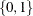, with 1 representing the event for which the probability is to be modeled. Consider the example where Y takes the value 1 for event and 0 for nonevent, and X is the explanatory variable. To specify the value 1 as the event category, use the following MODEL statement:

model Y (event='1') = X;

ORDER=order-type

specifies the sort order for the categories of categorical variables. This ordering determines which parameters in the model correspond to each level in the data. When the default ORDER=FORMATTED is in effect for numeric variables for which you have supplied no explicit format, the levels are ordered by their internal values. Table 24.4 shows how PROC ADAPTIVEREG interprets values of the ORDER= option.

Table 24.4: Sort Order for Categorical Variables

order-type

Levels Sorted By

DATA

Order of appearance in the input data set

FORMATTED

External formatted value, except for numeric variables with no explicit format, which are sorted by their unformatted (internal) value

FREQ

Descending frequency count; levels with the most observations come first in the order

FREQDATA

Order of descending frequency count, and within counts by order of appearance in the input data set when counts are tied

FREQFORMATTED

Order of descending frequency count, and within counts by formatted value (as above) when counts are tied

FREQINTERNAL

Order of descending frequency count, and within counts by unformatted value when counts are tied

INTERNAL

Unformatted value

For the FORMATTED and INTERNAL values, the sort order is machine-dependent. If you specify the ORDER= option in the MODEL statement and the ORDER= option in the CLASS statement, the former takes precedence.

For more information about sort order, see the chapter on the SORT procedure in the Base SAS Procedures Guide and the discussion of BY-group processing in SAS Language Reference: Concepts.

REFERENCE='category' |FIRST |LAST
REF='category' |FIRST |LAST

specifies the reference category for the binary or multinomial response model. For the binary response model, specifying one response category as the reference is the same as specifying the other response category as the event category. You can specify one of the following values for this option:

'category'

specifies the formatted value of the reference category.

FIRST

designates the first ordered category as the reference.

LAST

designates the last ordered category as the reference.

The default is REFERENCE=LAST.

#### Model Options

You can specify the following model options.

requests an additive model for which only main effects are included in the fitted model. If you do not specify the ADDITIVE option, PROC ADAPTIVEREG fits a model that has both main effects and two-way interaction terms.

ALPHA=number

specifies the parameter that controls the number of knots considered for each variable. Friedman (1991b) uses the following as the number of observations between interior knots: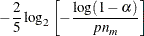Friedman also uses the following as the number of observations between extreme knots and the corresponding variable boundary values,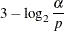where p is the number of variables and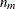is the number of observations for which a parent basis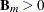. The value ofshould be greater than 0 and less than 1. The default is ALPHA=0.05.

CVMETHOD=RANDOM <(n)>
CVMETHOD=INDEX (variable)

specifies the method for subdividing the training data into n parts when you request n-fold cross validation when you do backward selection. CVMETHOD=RANDOM assigns each training observation randomly to one of the n parts. CVMETHOD=INDEX(variable) assigns observations to parts based on the formatted value of the named variable. This input data set variable is treated as a classification variable, and the number of parts n is the number of distinct levels of this variable. By optionally naming this variable in a CLASS statement, you can use the ORDER= option in the CLASS statement to control how this variable is levelized.

The value of n defaults to 5 with CVMETHOD=RANDOM.

DFPERBASIS=d
DF=d

specifies the degrees of freedom (d) that are charged for each basis function that is used in the lack-of-fit function for backward selection. Larger values of d lead to fewer spline knots and thus smoother function estimates. The default is DFPERBASIS=2.

DIST=distribution-id

specifies the distribution family used in the model.

If you do not specify a distribution-id, the ADAPTIVEREG procedure defaults to the normal distribution for continuous response variables and to the binary distribution for classification or character variables, unless the events/trial syntax is used in the MODEL statement. If you choose the events/trial syntax, the ADAPTIVEREG procedure defaults to the binomial distribution.

Table 24.5 lists the values of the DIST= option and the corresponding default link functions. For generalized linear models with these distributions, you can find expressions for the log-likelihood functions in the section Log-Likelihood Functions.

Table 24.5: Values of the DIST= Option

distribution-id

Aliases

Distribution

BINOMIAL

Binomial

Logit

GAMMA

GAM, G

Gamma

Reciprocal

GAUSSIAN

NORMAL, N, NOR

Normal

Identity

IGAUSSIAN

IG

Inverse Gaussian

Inverse squared

(power(–2))

NEGBIN

NB

Negative binomial

Log

POISSON

POI

Poisson

Log

FAST<(fast-options)>

improves the speed of the modeling. Because of the computation complexity in the original multivariate adaptive regression splines algorithm, Friedman (1993) proposes modifications to improve the speed by tuning several parameters. See the section Fast Algorithm for more information about the improvement of the multivariate adaptive regression splines algorithm. You can specify the following fast-options:

BETA=beta

specifies the aging factor in the priority queue of candidate parent bases. Larger values of beta result in low-improvement parents rising fast into top list of candidates. The default value is BETA=1.

H=h

specifies the parameter that controls how often the improvement is recomputed for a parent basis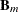over all candidate variables. Larger values of h cause fewer computations of improvement. The default value is H=1.

K=k

specifies the number of top candidates in the priority queue of parent bases for selecting new bases. Larger values of k cause more parent bases to be considered. The default is to use all eligible parent bases at every iteration.

FORWARDONLY

skips the backward selection step after forward selection is finished.

KEEP=effects

specifies a list of variables to be included in the final model.

LINEAR=effects

specifies a list of variables to be considered without nonparametric transformation. They should appear in the linear form if they are selected.

specifies the link function in the model. Not all link functions are available for all distribution families. The keywords and expressions for the associated link functions are shown in Table 24.6.

keyword

Alias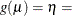CLOGLOG

CLL

Complementary log-log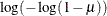IDENTITY

ID

Identity
LOG

Log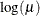LOGIT

Logit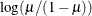POWERMINUS2

Power with exponent –2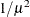PROBIT

NORMIT

Probit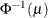RECIPROCAL

INVERSE

Reciprocal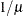MAXBASIS=number

specifies the maximum number of basis functions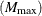that can be used in the final model. The default value is the larger value between 21 and one plus two times the number of nonintercept effects specified in the MODEL statement.

MAXORDER=number

specifies the maximum interaction levels for effects that could potentially enter the model. The default value is MAXORDER=2.

NOMISS

excludes all observations with missing values from the model fitting. By default, the ADAPTIVEREG procedure takes the missingness into account when an explanatory variable has missing values. For more information about how PROC ADAPTIVEREG handles missing values, see the section Missing Values.

OFFSET=variable

specifies an offset for the linear predictor. An offset plays the role of a predictor whose coefficient is known to be 1. For example, you can use an offset in a Poisson model when counts have been obtained in time intervals of different lengths. With a log link function, you can model the counts as Poisson variables with the logarithm of the time interval as the offset variable. The offset variable cannot appear in the CLASS statement or elsewhere in the MODEL statement.

VARPENALTY=specifies the incremental penaltyfor increasing the number of variables in the adaptive regression model. To discourage a model with too many variables, at each iteration of the forward selection the model improvement is reduced by a factor of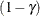for any new variable that is introduced.

For highly collinear designs, the VARPENALTY= option helps PROC ADAPTIVEREG produce models that are nearly equivalent in terms of residual sum of squares but have fewer independent variables. Friedman (1991b) suggests the following values for:

0.0

no penalty (default value)

0.05

moderate penalty

0.1

heavy penalty

The best value depends on the specific situation. Some experimenting with different values is usually required. You should use this option with care.